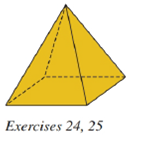Chapter 9.2, Problem 25E### Elementary Geometry for College St...

6th Edition
Daniel C. Alexander + 1 other
ISBN: 9781285195698

#### Solutions

Chapter
Section### Elementary Geometry for College St...

6th Edition
Daniel C. Alexander + 1 other
ISBN: 9781285195698
Textbook Problem
1 views

# For the regular square pyramid shown in Exercise 24, suppose that the sides of the square base measure 6 f t each and that the altitude measures 4 f t in length.a) Find the lateral area L of the pyramid.b) Find the total area T of the pyramid.c) Find the volume V of the pyramid.To determine

(a)

To find:

The lateral area of the pyramid.

Explanation

Given:

A regular square pyramid shown, suppose that the sides of the square base measure 6ft each and that the altitude measures 4ft.

Properties used:

A pyramid is made by connecting a base to an apex. The base is flat with straight edges, no curves, hence, a polygon and all other faces are triangles.

A regular pyramid is a pyramid whose base is a regular polygon and whose lateral edges are all congruent.

The lateral area L of a regular pyramid with slant height of length l and perimeter P of the base is given by

L=12lP.

In a regular pyramid, the lengths of the apothem a of the base, the altitude h, and the slant height l satisfy the Pythagorean Theorem; that is, l2=a2+h2.

The perimeter of the square is four times the side.

Calculation:

From the given figure,

The sides of the square base measure 6ft each and that altitude measures 4ft i.e. h=4ft.

Since, the apothem is half the base length. Hence, a=62=3ft

To determine

(b)

To find:

The total area of the pyramid.

To determine

(c)

To find:

The volume of the pyramid.

### Still sussing out bartleby?

Check out a sample textbook solution.

See a sample solution

#### The Solution to Your Study Problems

Bartleby provides explanations to thousands of textbook problems written by our experts, many with advanced degrees!

Get Started

#### In Exercises 21-24, find the distance between the given points. 21. (1, 3) and (4, 7)

Applied Calculus for the Managerial, Life, and Social Sciences: A Brief Approach

#### In Problems 1-20, evaluate the improper integrals that converge.

Mathematical Applications for the Management, Life, and Social Sciences

#### Sometime, Always, or Never:

Study Guide for Stewart's Single Variable Calculus: Early Transcendentals, 8th

#### The graph at the right has equation:

Study Guide for Stewart's Multivariable Calculus, 8th